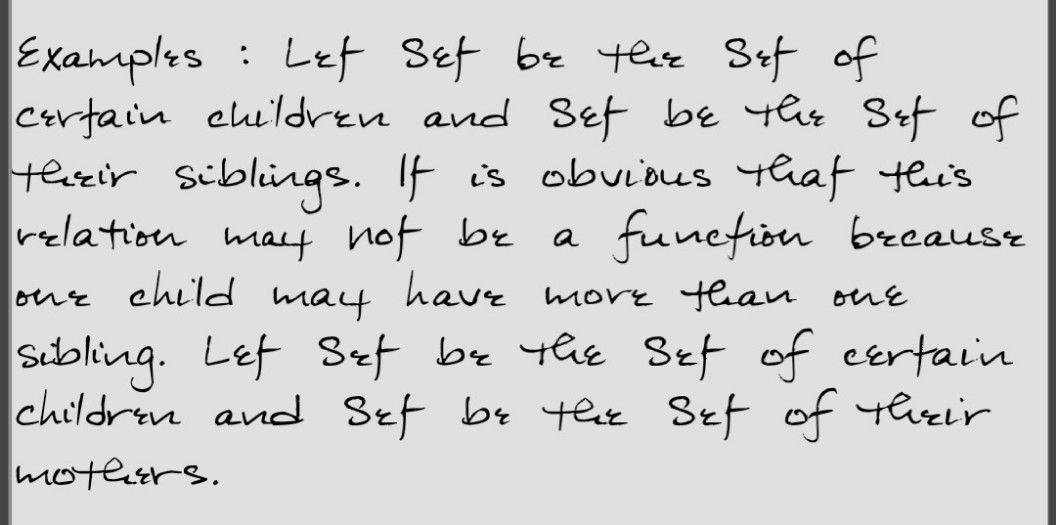Symbol
ProblemWhat $l$ Can $\square 0$ Activity $3$ Apply Me $1.$ Cite a $real-wo\pi d$ example or scenario that can be expressed as a relation that is not a function. $2.$ Cite a $\pi eal-wo\pi Q$ scenario of a scenario that can $be$ modeled $by$ a linear function. 3. A videoke machine can be rented for $P1,000.00$ for three $days$ $bM1$ on the fourth day and onwards, an additional $cost$ of P400 $Perday$ $M\vec{1} 1\right)$ be added to the rent. Represent the $cos1$ of renting a videoke machine as a $D1ecew1s8$ function of the number of $1\square$ days it is rented and plot its graphs,
10th-13th grade
Other
Search count: 105
SolutionQanda teacher - AskhinaThanks for asking a question. You can ask any doubt without any hesitation in a direct way also for high-quality answers quickly and please rate 5 stars if you are satisfied with my answers And, if you have no doubt related to the previous question then please evaluate the answer and give 3000 coins as a gift ( these are not reduced to your wallet)